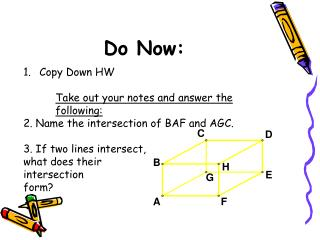# Do Now: - PowerPoint PPT PresentationDownload PresentationDo Now:

Do Now:
Download Presentation## Do Now:

- - - - - - - - - - - - - - - - - - - - - - - - - - - E N D - - - - - - - - - - - - - - - - - - - - - - - - - - -
##### Presentation Transcript

1. Do Now: • Copy Down HW • Take out your notes and answer the following: • 2. Name the intersection of BAF and AGC. • 3. If two lines intersect, • what does their • intersection • form?

2. Ch. 1.2 Use Segments and Congruence 2 cards

3. Definition A segment is made up of the line in between two points, excepts segments do not continue on forever. B A

4. Ruler Postulate The distance between any two points is the absolute value of the difference of the corresponding numbers

5. Congruent Segments Segments with the same length

6. Segment Addition Postulate If 3 points, A, B and C are collinear and B is between A and C, then AB + BC = AC

7. Ex 1:Plot the points in a coordinate plane and determine whether the line segments are congruent

8. Example 2: in the diagram, HE=12 miles, and HS=17 miles. How long is ES? H E S

9. Example 3: In the diagram, SA=2x-1, AT=3x+4, and ST=10. Find x then find the length of SA. T A S

10. Let’s Go Outside! Geometry Photo Scavenger Hunt • In groups of two, you will go outside and find and take pictures of the following: • A segment between two points • Two intersecting lines • Two intersecting planes • A line intersecting a plane • Two lines that do not intersect • Two planes that do not intersect

11. Let’s Go Outside! Geometry Photo Scavenger Hunt Once you take pictures of all the items on the list, send them to me in an email: joyk@villagechristian.org First two groups to send me an email with all items get a prize!

12. Exit Slip: In the diagram, the points A,B,C and D are collinear. AD=60, AB=14, and BC=CD Find CD